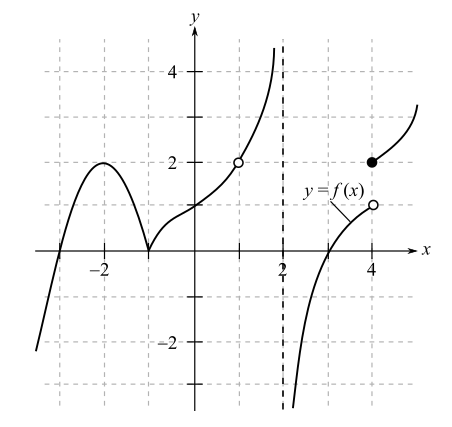Chapter 9, Problem 45RE### Mathematical Applications for the ...

11th Edition
Ronald J. Harshbarger + 1 other
ISBN: 9781305108042

#### Solutions

Chapter
Section### Mathematical Applications for the ...

11th Edition
Ronald J. Harshbarger + 1 other
ISBN: 9781305108042
Textbook Problem

# Use the graph of y = f(x) in Figure 9.40 on the previous page to answer the questions in Problems 44-46.Is f(x) differentiable at ( a )   x   =   − 1 ?       ( b )   x   = 1 ?

(a)

To determine

Whether the function with the following graph is differentiable at x=1,Explanation

Given Information:

The provided graph of the function is,

Explanation:

Consider the provided graph of function,

(b)

To determine

Whether the function with the following graph is differentiable at x=1,### Still sussing out bartleby?

Check out a sample textbook solution.

See a sample solution

#### The Solution to Your Study Problems

Bartleby provides explanations to thousands of textbook problems written by our experts, many with advanced degrees!

Get Started

#### In Exercises 4562, find the values of x that satisfy the inequality (inequalities). 46. 6 4 + 5x

Applied Calculus for the Managerial, Life, and Social Sciences: A Brief Approach

#### Find the derivative of the function. g(x) = (x2 + 1)3(x2 + 2)6

Single Variable Calculus: Early Transcendentals, Volume I

#### Evaluate . e + 1

Study Guide for Stewart's Single Variable Calculus: Early Transcendentals, 8th